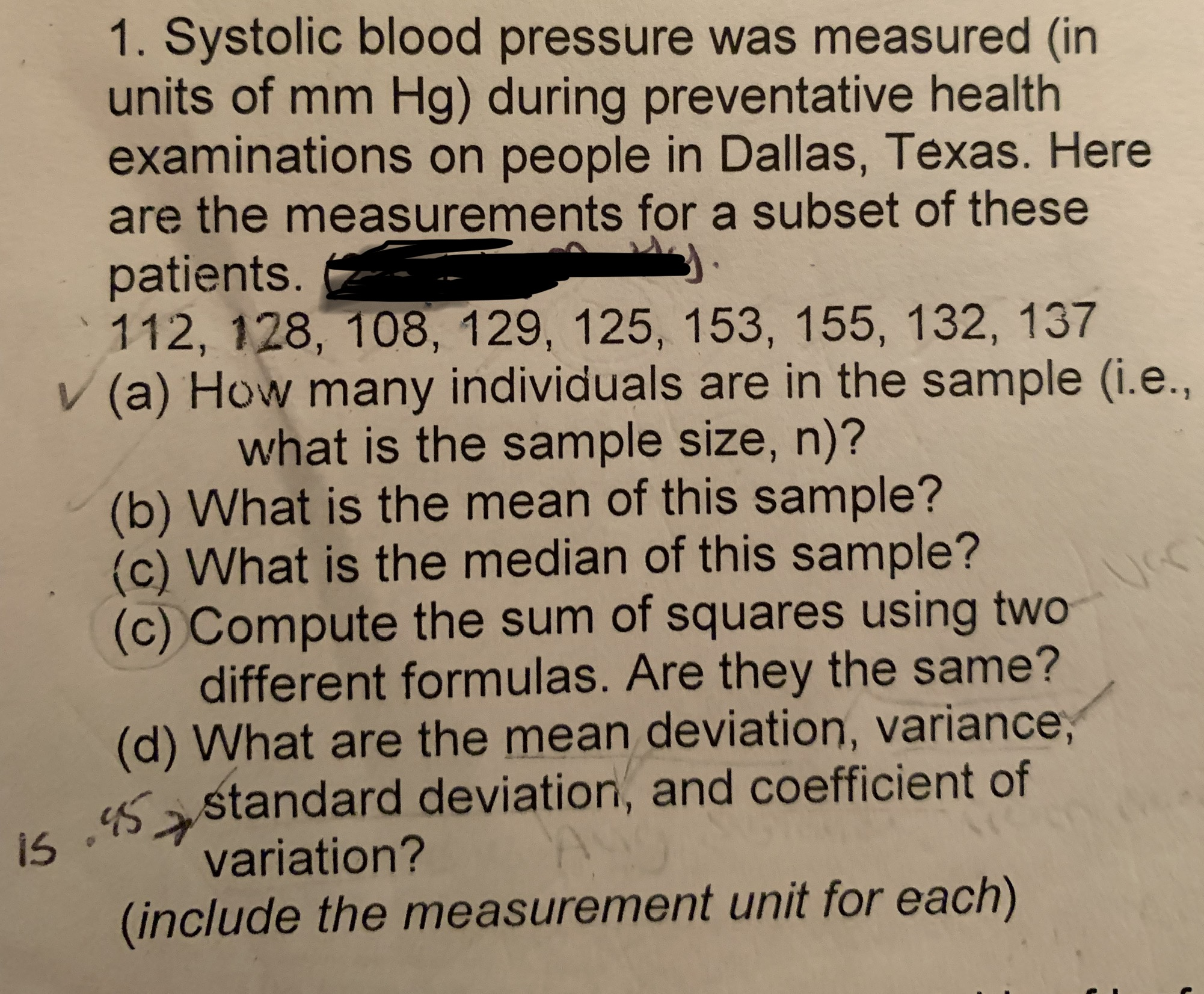1. Systolic blood pressure was measured (inunits of mm Hg) during preventative healthexaminations on people in Dallas, Texas. Hereare the measurements for a subset of thesepatients.112, 128, 108, 129, 125, 153, 155, 132, 137V (a) How many individuals are in the sample (i.e.,what is the sample size, n)?(b) What is the mean of this sample?(c) What is the median of this sample?(c) Compute the sum of squares using twodifferent formulas. Are they the same?(d) What are the mean deviation, variance,s astandard deviation, and coefficient ofvariation?(include the measurement unit for each)

Questionhelp_outlineImage Transcriptionclose1. Systolic blood pressure was measured (in units of mm Hg) during preventative health examinations on people in Dallas, Texas. Here are the measurements for a subset of these patients. 112, 128, 108, 129, 125, 153, 155, 132, 137 V (a) How many individuals are in the sample (i.e., what is the sample size, n)? (b) What is the mean of this sample? (c) What is the median of this sample? (c) Compute the sum of squares using two different formulas. Are they the same? (d) What are the mean deviation, variance, s astandard deviation, and coefficient of variation? (include the measurement unit for each) fullscreen
Step 1

Blood pressure is measured in units of "millimetres...

Want to see the full answer?

See Solution

Want to see this answer and more?

Our solutions are written by experts, many with advanced degrees, and available 24/7

See Solution
Tagged in

Animal Physiology and Biochemistry